﻿ Stokes parameters in B-mode CMB polarization and gravitational-waves.

# Cosmic inflation is "fantasy"

## Reason why cosmic inflation is wrong.

(Fig.1) Faster-than-light universe expansion = inflation is real ?   or "illusion" ?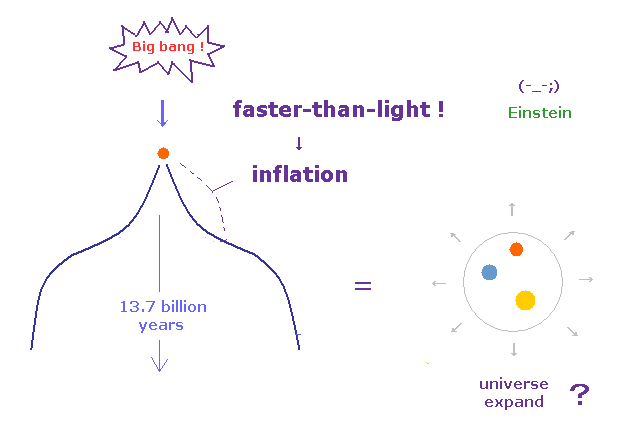The recent news say the proof of cosmic inflation and gravitational waves have been found , as shown in various webnews ( this, this, this, this ).

But unfortunately, it is impossible to conclude that "fantasy" inflation actually happened ONLY from this indirect observation.

"Inflation" means our universe expanded much faster-than-light just after ( or before ) Big Bang.
And it is quite unnatural that very faint polarization (= B-mode, only 0.4 μK ! ) about 13.7 billion years ago, remains intact as it is until now.

## Very powerful "Inflaton" really existed ?

(Fig.2) "Inflaton" caused faster-than-inflation !?   What is "Inflaton" !?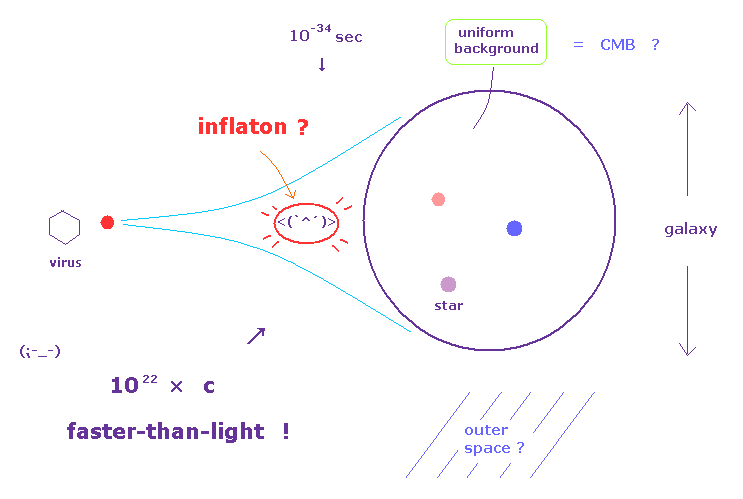As shown on this page, during very short time of 10-34 seconds just after Big Bang, very tiny space like a virus expanded to that as large as "galaxy".

So this inflation speed reached more than 1022 times the speed of light !
They try to explain this unrealistic inflation using artificial particles of "inflaton".
Of course, this inflaton is only speculative concept, which cannot be detected at all.

Bloggers and web (TV) news sites just blindly believe (← NOT science but "religion" ) this "fantasy" inflation.
This tendency is very harmful for "gullible" ordinary people.
Physicsworld is better than other webnews sites, because some opposing opinions are seen.

## Horizon problem (= faster-than-light relation ! ) proves Big Bang theory is wrong.

(Fig.3) Cosmic microwave background (= CMB ) is uniform among faster-than-light area !?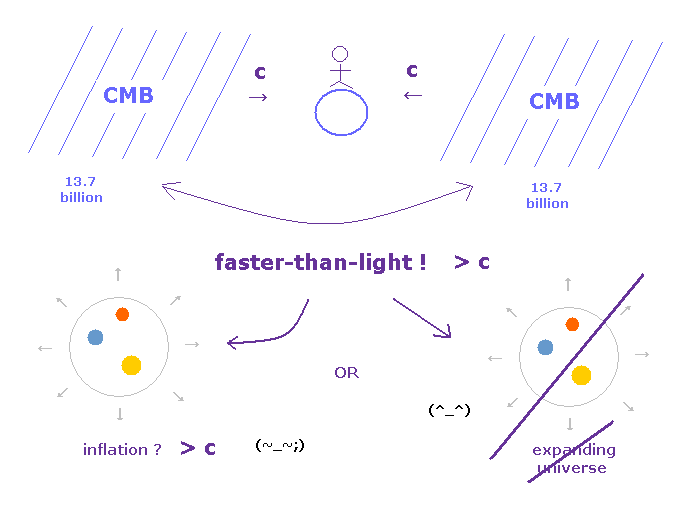This "ad-hoc" inflation theory was introduced to explain horizon problem in uniform cosmic microwave background (= CMB ).
According to the current Big Bang (= expanding universe ) theory, our universe is only 13.7 billion years old.
Considering the maximum light speed "c", the light (= microwave ) cannot go beyond 13.7 billion light-years area ( see also this site ).

But this CMB (= microwave ) from the opposite sides (= 13.7 × 2 = 27.4 billion ) is also uniform, so some faster-than-light interaction is indispensable for this homogeneity (= horizon problem ), they insist.

As you notice, if we reject the ad-hoc expanding universe, the limit age of 13.7 billion can be removed, so faster-than-light relation does NOT need to be used.
As shown on this page, we can naturally explain uniform CMB using ether-like matter and tired-light theory.

## Dark energy, dark matter, Higgs, virtual particles = ether revived ?

(Fig.4) Ether was denied → virtual particles, dark matter, energy, Higgs.. = ether ??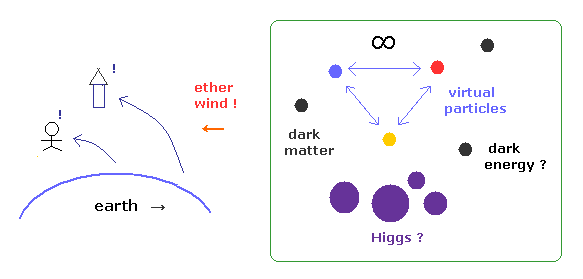Though they rejected "ether", relativistic quantum field theories need infinite virtual particles, dark matter, dark energy and Higgs filling all the vacuum.
This idea is completely inconsistent with special relativity, which denied "ether".
In fact, the kinds of "ether" have been increased much more in relativisitic quantum field theory !

And as shown on this page, special realtivity includes fatal paradoxes (= Ehrenfest, trouton-noble, right-angle lever.. )
If we accept one kind of "ether"-like matter from the beginning, we can explain uniform cosmic microwave background (= CMB ) and dark matter , without artificially increasing the kinds of ether.

And as I said many times, if various academic organizations are hiding fatal paradoxes of relativity from students, it is clearly one of frauds, vicious crimes.

## Accepting inflation means fantasy "multiverse" is right !?

(Fig.5) Inflation = "fantasy" multiverse and 10 dimensional string theory.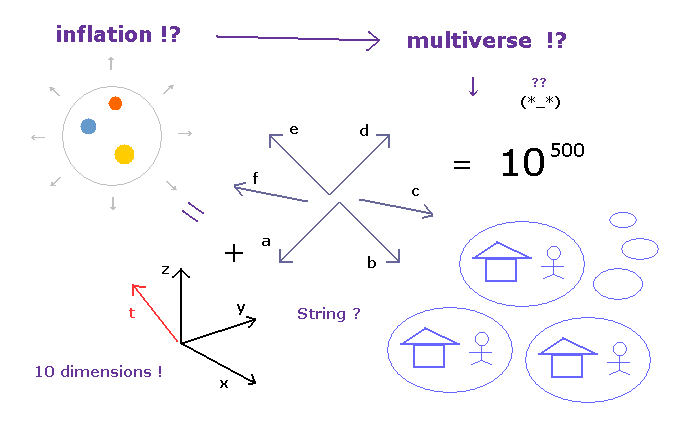If faster-than-light expansion of our univserse is accepted, we need to believe "fantasy" multiverse (= many-worlds ) and 10-dimensional string theory.
But as most people feel, these multiverse and extradimensions are completely out of touch with reality.
So "inflation" itself cannot be accepted at all.

As shown in this news and this news, they argue, when the universe inflated faster than the speed of light, the expansion ripped the vacuum of spacetime into separate pieces, or multiple universes.
Can you really believe this ad-hoc theory ( = "fantasy" ) ?   Probably NOT.

### [ Spacetime itself moves faster-than-light ? ← Special relativity is NOT "universal" theory ? ]

(Fig.6) Our universe ( and "inflaton" ) can move faster-than-light through outer space !?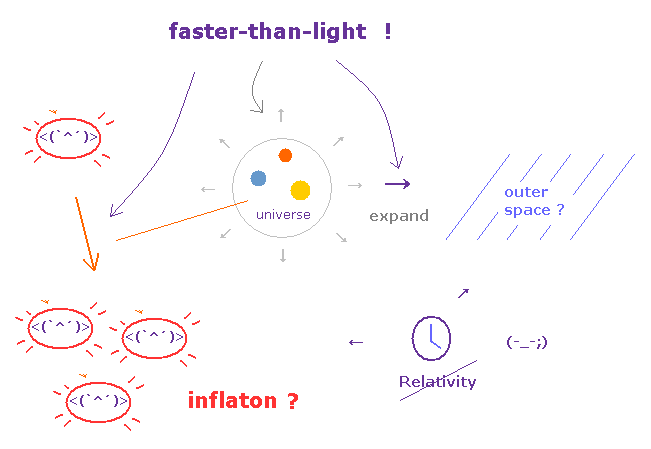According to this site and this site, even if the spacetime itself moves faster than light, there is no violation of special relativity.
( Only if some information propagates faster-than-light, it's violation, they insist. )

But this logic is very artificial and too good to be true.
If this inflation is true, our universe ( and "inflaton" particles ) can move faster-than-light with respect to outer space around the universe.

And what is the boundary between our universe and outer space made of ?
Why this boudary doesn't let anything through, even when it is stretched ? This is strange.

## CMB = ether !? = Newton absolute space ?

(Fig.7) The earth is moving with respect to CMB (= ether !? ) at 400 km/s !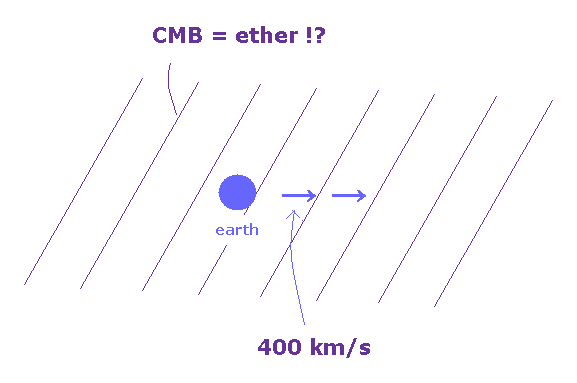Of course, in relativistic world, the concept of Newton's absolute space (= ether ) is inhibited.
But observation of CMB indicates the earth is actually moving through CMB at the velocity of 400 km/s, as shown on this site, this site and this site.

This speed is faster than orbital speed (= 30 km/s ) of the earth around the sun.
CMB is uniform microwave background filling all over the universe.
This anisotropy in CMB spectrum clearly shows CMB is "ether" like matter.

And this result is completely consistent with "earth ether" moving with the earth, as shown in Michelson-Morley experiment.
( Inside this earth ether, light speed is constant "c". )

## CMB is realy "imprint" of inflation 13.7 billion years ago !?

(Fig.8) Very faint B-mode polarization is really left as it is, during 13.7 billion years !?As most people feel, it is impossible that very faint ( CMB ) light polarization is left as it is, during as long as 13.7 billion years.
This is clearly fatal defect in this Big Bang theory.

But various bloggers, webnews sites and even top journals ( Nature, Science ) do NOT try to refer to this fatal defect. This is very strange.
So at this moment, these things are NOT science, but just some "religion".

As you see the disastrous fact that 10-dimensional string theory is dominant in the present physics, the basis of physics is completely distorted, because of wrong assumtions (= many-worlds, relativity ).

## What caused the present B-mode polarization ? → Only inflation !?

(Fig.9) Question: What caused B-mode ? → 1. inflation, 2. inflation, 3. inflation ... !?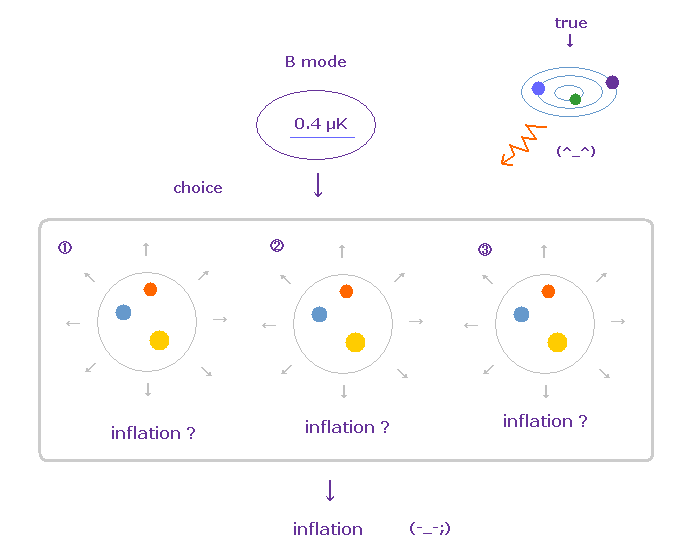As you see various webnews sites, they too easily jump to the conclusion that the curling pattern of light polarization proves the inflation in early universe.
But the observed CMB IS the current ( NOT early universe ! ) microwave.

The most serious problem is that even academic organizations do NOT try to refer to other more probable reasons such as various radiation from infinite stars and space matters during extremely long period.

These problematic attitudes are very similar to those blindly believing spin, even though weak spin is inconsistent with experimental results.
It's like a failure of some detective stories, in which police is chasing ONLY one suspect, from the beginning.

## CMB really means the light about 400000 years after Big Bang ?

(Fig.10) The state (= polarization ) of CMB is the same as that of early universe !?   Really ?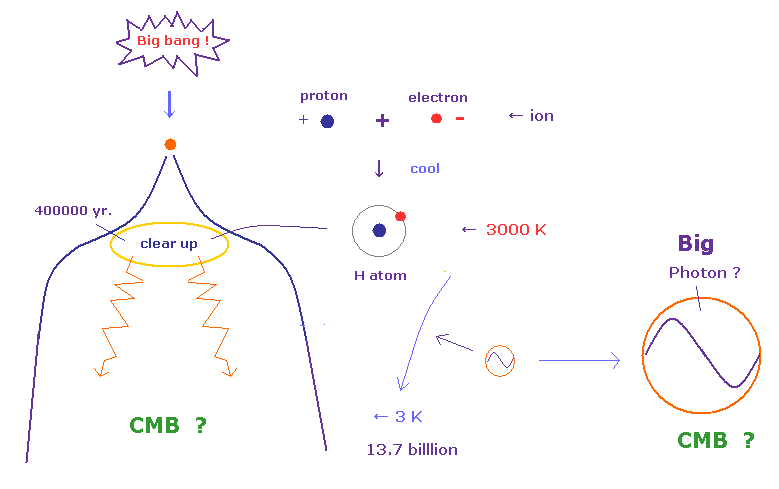The universe began with Big Bang 13.7 billion years ago.
Faster-than-light inflation followed immediately, which produced high-amplitude gravitational wave, they insist.

According to this site and this site, about 400000 years after Big Bang, the universe expanded and cooled enough for protons and electrons to form neutral hydrogen, opening up a path for light to travel freely.
That first light persists today as the CMB, which has been stretched to microwave wavelength.

At that time (= 400000 years after Big Bang ), the temperature is as high as 3000 K, which means very fast fluctuation of lights.
So it is natural that this very fast fluctation at 3000 K cancelled out faint polarization information at that time.

## Why ONLY "photon" particle was stretched by Big Bang ??

(Fig.11) Electron remains as it is. Why ONLY photon particle becomes "Big photon" ??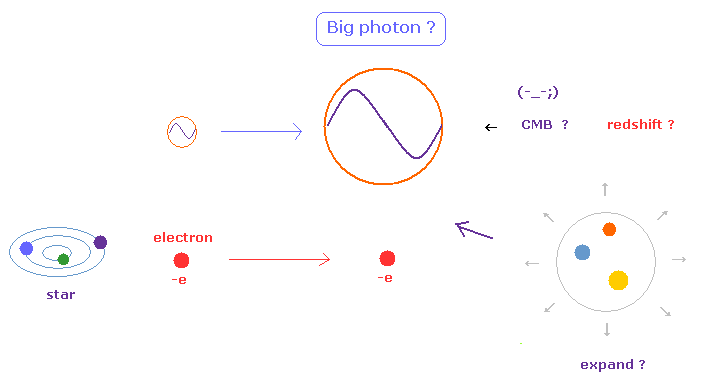The present Big Bang theory is based on the idea that cosmological redshift is due to the elongation of "photon" by the universe expansion.
This means only point-like photon becomes much bigger (= Big photon ) ?
Though this big particle have NOT been observed.

Of course, if all particles included in the universe expanded in the same way, we could NOT notice the universe is expanding.

As you know, the quantum mechanics insists all particles such as electrons and protons have wave-like properties (= de Broglie wave ).
Then why other particles such as electrons, protons, various atoms and molecules did NOT expand, though photon particle did. (= Photon is NOT a particle ?? )
So the present ad-hoc Big Bang theory includes self-contradiction from the beginning.

## The last scattering of CMB, 400000 years after Big Bang ?

(Fig.12) CMB lights are scatterd (= last scattering ) about 400000 years after Big Bang ?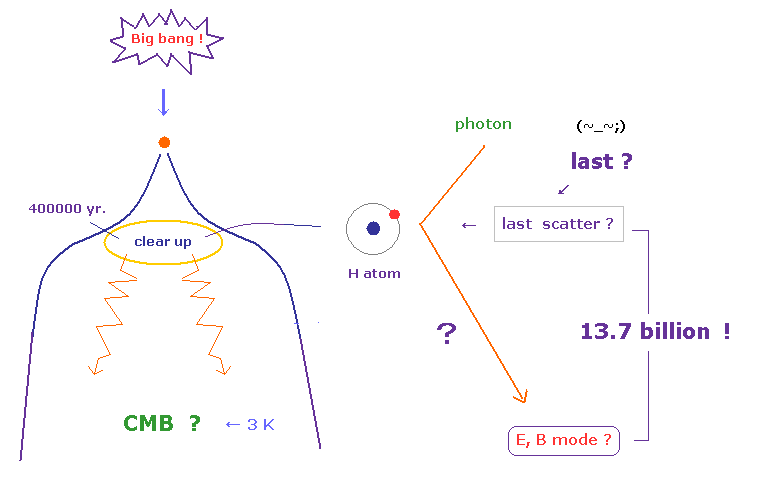As shown on this site and this site, the lights experienced last scattering about 400000 years after Big Bang.
This last scattering was basically ( classical ) Thomson scattering, which generated E-mode polarization.
If there are some gravitaional waves generated at inflation, this polarization is distorted, to be B-mode.

But this explanation relies on too idealistic assumtions .
It is natural that the spase at that time had already contained infinite kinds of polarization patterns.
So B-mode pattern (= curl ) can be caused by combination of natural ( NOT gravity wave ) scattering.

## CMB could really survive through reionization period !?

(Fig.13) CMB polarization pattern in early universe are really preserved ?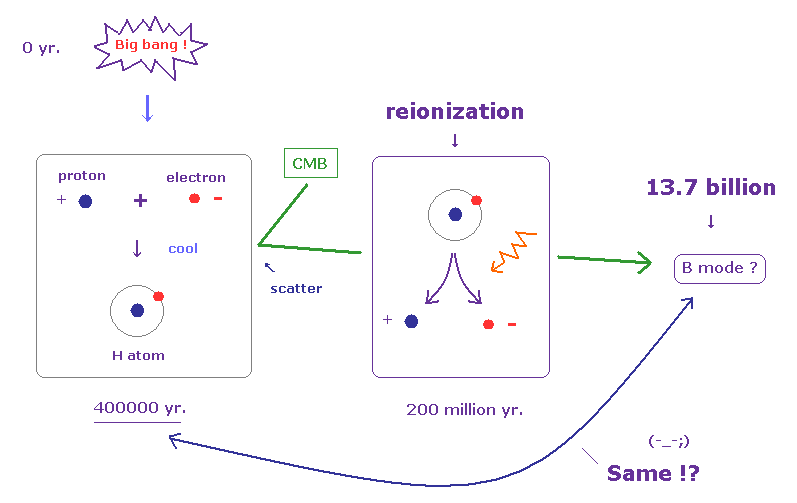But as you see the cosmic rays in the current universe, almost all atoms are ionized into protons and electrons flying at light speed.
So they insist neutral atoms experienced reionization (= about 200 million years after Big Bang ), in which some high energy source ionized all atoms.

Do you think this Big Bang theory is too good to be true ?
If there were so high energy sources, it is inconsistent with the formation of neutral atoms before that, when CMB is emitted.

And it is impossible that faint CMB polarization keeps as it is, even in these strict circumstances, which is filled with high energy sources.

## Again, it is impossible that faint CMB polarization is left as it is, for 13.7 billion years.

(Fig.14) CMB polarization can survive for as long as 13.7 billion years ? ← Impossible !As a result, from realistic (= scientific ) viewpoints, it is completely impossible that very faint CMB polarisation can keep its information "intact" for as long as 13.7 billion years.
So the current cosmic microwave background can NOT prove the existence of "fantasy" inflation at all.

## The current physics is filled with many "fantasy" concepts.

(Fig.15) Faster-than-light inflation, 10-dimensional string theory .. ← Physics ??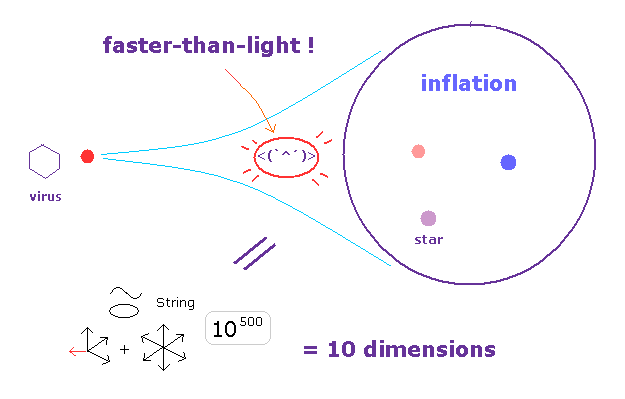So, believing this inflation is as unrealistic as believing 10-dimensional string theory.
The physics was completely distorted in the wrong direction in 1920s.

The current physicists are too familiar with imaginary ideas.
This is disastrous for development of science.

## Reason why "gravitational wave" does NOT exist.

### [ Pseudotensors of gravitaional waves are NOT unique ! ]

(Fig.16) Equivalence principle → Gravitational waves are cancelled out !?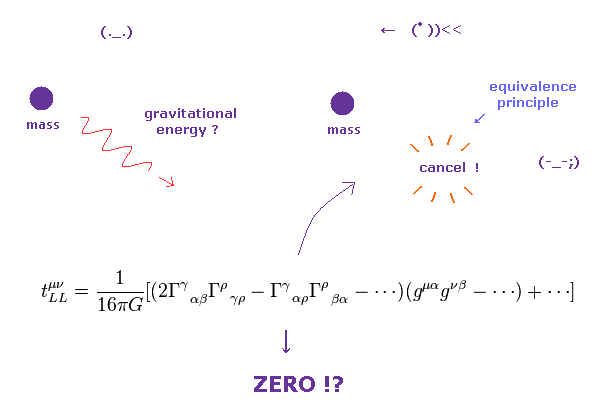It is said that the B-mode polarization and pulsar indirectly proved the existence of gravitational waves.
But in fact, this gravitational waves are completely "fake" waves.
Tensors describing gravitaional waves are NOT true tensors (= pseudotensor ).

There are two fatal defects in this gravitational pseudotensors.
One is, that the form of this pseudotensors are NOT unique.
So, we can artificially choose some convenient pseudotensor for experimental results.
Two is, that this energy-momentum pseudotensors completely vanish in some coordinate, which contradicts relativity.

On this site p.2 and this site p.5,
-----------------------------------------------
The disadvantage of pseudotensor tαβ is that it is NOT unique.
Various forms have been proposed for tαβ, each being based on certain specific consideration ....
There are many possible stress-energy tensors and pseudotensors.
----------------------------------------------

On this site (p.2) and this site (p.2-3).
------------------------------------------------
Gravitaional waves, pseudotendors globally vanish in some coodinates.
In consequence, they give in these coordinates "NO gravitational energy and energy flux".

It is important that the quantities tik do NOT constitute a tensor. They depend on the choice of coordinates. There are many suggestions for the pseudo-tensor
-------------------------------------------------

(Fig.17) "21000" light-years away ! → Can you believe this result ??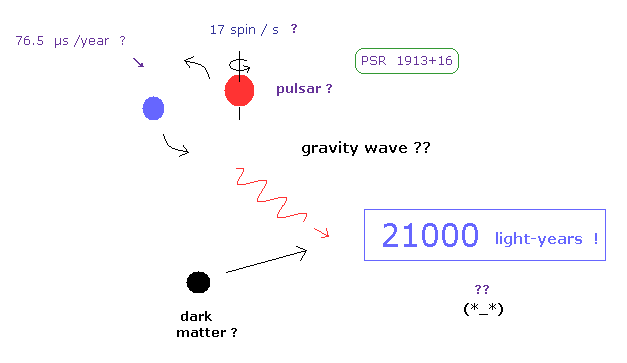They insist gravitational waves were indirectly proved by observing the orbital period change of pulsar 1913+16.
General relativity predicts this orbital period decreases by 76.5 microseconds per year. ( ← very small ! )

The problem is that this neutron star is as long as 21000 light-years away from the earth, as shown on this site.
It is much more impossible that we can predict accurately all influences caused by unknown things such as dark matter, quantum fluctuations included in 21000 light-years path !

So this test of general relativity is very doubtful.
It is natural these very slight change is due to some space matter's oscillation along the extremely long way.

## B-mode is too weak (= 0.4 μK ! ), cannot survive for 13.7 billion years.

(Fig.18) B-mode polarization is too weak (= 0.4 microK ) with respect to CMB (= 3K ).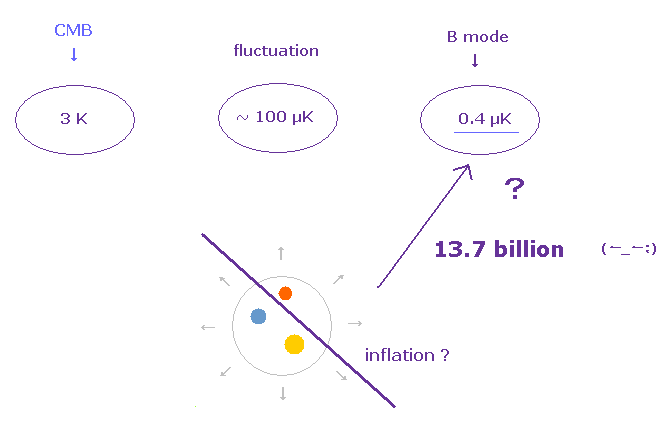As shown on this site, B-model polarization is very weak (= about 0.4 × 10-6 Kelvin ! ).
So it is extremely difficult to distinguish them from various other radiations.
As shown on this site, this site, this site, other polarization such as E-mode is about 100 μK.

So from the realistic viewpoint, B-mode curling polarization represents only faint lights emitted from stars or something ( NOT early universe ).
This means primordial gravitational waves are unnecessary.

## What are "E, B- mode" polarization ? ← very vague concept.

(Fig.19) E-mode polarization ?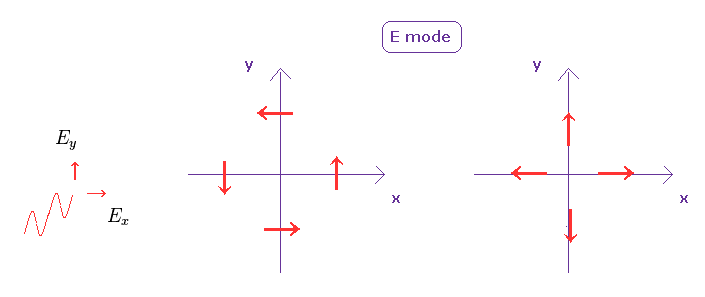As shown on this site and this site, "E-mode" polarization is parallel or perpendicular to wave vector k.
And B-mode polarization is oriented at ±45o to vector k.

The important point is that the cosmic microwave background is a collective lights of various wavelength and polarization ( NOT single photon ).
So it is quite natural that some slight porization asymmetry is seen inside unpolarized lights

(Fig.20) B-mode polarization ?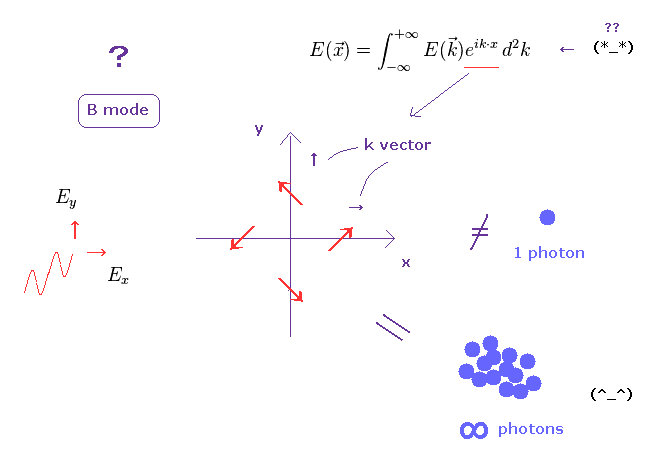On this site,
------------------------
Take, for example, the radiation incident onto the electron from the left to be more intense than the radiation incident from the right, with average intensities ( of scatterd lights ) above and below (that's a dipole); it then suffices to sum up all contributions to see that no net polarization survives.
-------------------------
So the current CMB theory is based on average unpolarized lights in the early universe.
This condition is too special and too idealistic.

The problem is the wave vector k is also vague concept.
They do Fourier expansion on polarization distribution.
So this wave vector is arbitrary, which means various effects other than gravitational waves can explain B-mode.

## E, B mode by Stokes parameter.

(Fig.21) Light waves.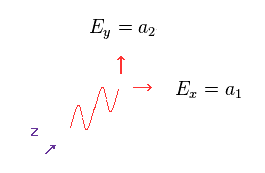In this section, we represent polarization of E and B mode using Stokes parameters ( see this site, this site ).
In Fig.21, some electromagnetic wave is propagating in the (-)z direction.
So the polarization (= electric field ) must be in the x-y plane.

(Eq.1)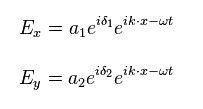We could therefore break down the electric field into x and y components, as shown in Eq.1
"a1" and "a2" are light amplitude in x and y directions, respectively.

(Eq.2)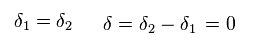Here we consider simple linear ( not elliptical ) polarization.
So the phase difference (= δ ) between x and y directions are zero, as shown in Eq.2.

Here we define the following Stokes parameters,
(Eq.3)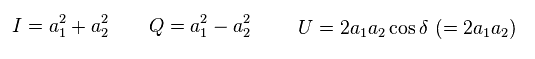where δ = 0.
And we can perform Fourier transformation on each parameter.
( So this wavevector is very "vague" and "arbitrary" mathematical concepts. )

(Eq.4)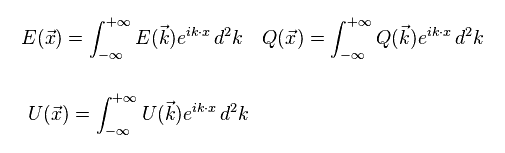We determine the parameters with respect to arbitrary wavevector k.
The parameters of Q and U in Eq.3 change under the rotation in x-y plane.

So we need to think about the rotated coodinate in which some "k" vector turns in new x' direction.
( The angle between this k vector and the original x axis is "φ" )
In this coodinate, a1 and a2 transform like
(Eq.5)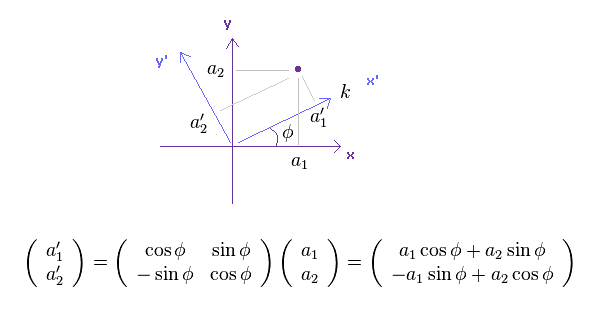Using Eq.5 and Eq.3, new Q parameter in this x'-y' plane becomes
(Eq.6)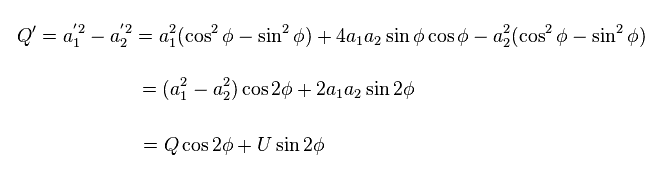where we use
(Eq.7)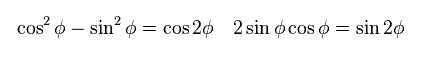And new U parameter in x'-y' plane becomes
(Eq.8)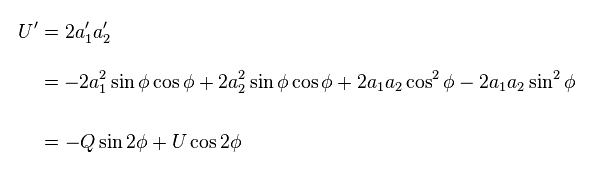These new Q' and U' in x'-y' plane ( in which arbitrary k vector is in x' direction ) are called "E-mode" and "B-mode", respectively.

(Eq.9)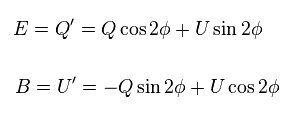When we consider the case in which the angle φ = 0.
( So, k wave vector is in x direction from the beginning ).

Substituting φ=0 into Eq.9,
(Eq.10)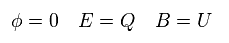When E-mode is NOT zero, and B-mode is zero,
(Eq.11)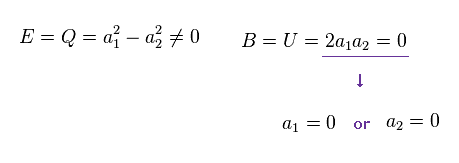When B-mode is zero, either of a1 or a2 becomes zero.
(Eq.12) E-mode polarization.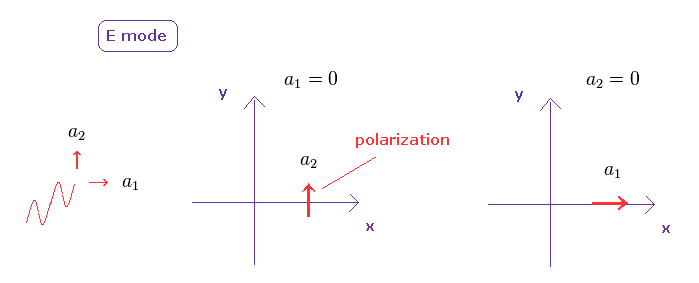As shown in Eq.12, in E-mode, polarization is parallel ot perpendicular to x axis (= k vector ).

(Eq.13) B-mode.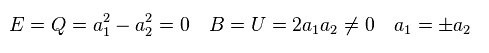When only E-mode is zero, absolute value of a1 and a2 become the same.

(Eq.14) B-mode polarization.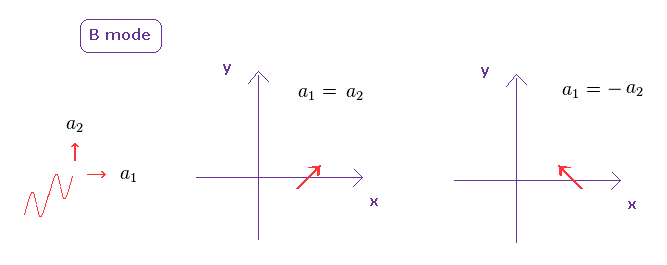As shown in Eq.14, B-mode polarization is oriented at ±45o to x axis (= k vector ).
When we consider all direction of k vector, E and B mode polarizations become

(Eq.15) E-mode polarization.(Eq.16) B-mode polarization.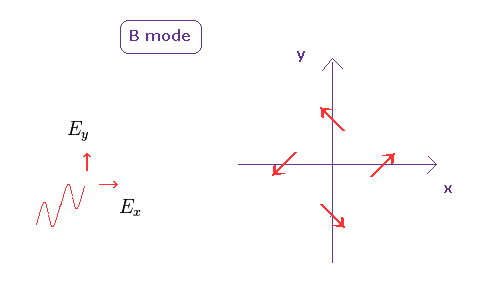## Thomson scattering about 13.7 billion years ago is really the "last" scattering ??

(Eq.17) Thomson scattering = classical mechanics.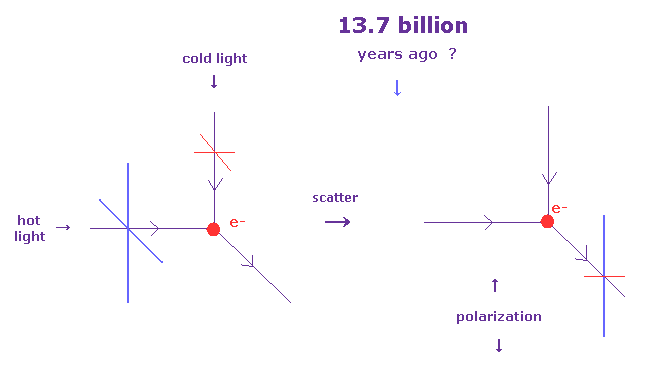According to the inflation theory, the current CMB polarization is the result of the last scattering about 13.7 billion years ago.
( This precondition is impossible from the realistic viewpoint. ).

As shown on this site and this site, when low-amplitude (= cold ) light comes from upper, and high-amplitude (= hot ) light comes from the left.

The net amplitude of light scattered ( by shaking electron ) becomes vertically polarized, as shown in Eq.17.
Basically, they don't think about various polarization patterns and compilicated scattering in this infation theory. So this assumption is too idealistic and too simple.

## Parameters of total scattered lights from all directions.

(Eq.18)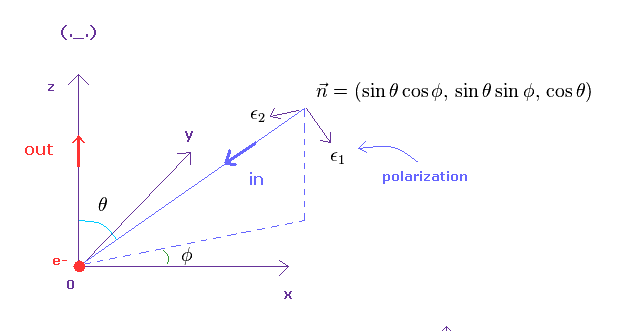Here we calculate Stokes parameter U and P of total scatterd lights from all directions.
In Eq.18, some incident light comes toward the electron at the origin in "-n" direction.

(Eq.19)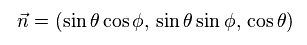ε1 and ε2 are unit vectors which are perpendicular to "n" vector.
So this insident light is polarized in these directions.

ε1 vector is in the same plane including both "n" and z axis.
And ε2 is perpendicular to this ε1 vector.

(Eq.20)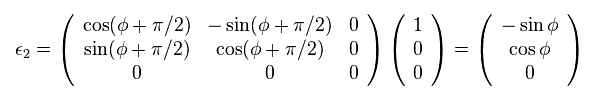ε2 vector can be gotten by rotating x unit vector by φ+π/2 on x-y plane.

To get ε1, we first rotate x unit vector by -θ in x-z plane.
And then we rotate it by φ in x-y plane.

(Eq.21)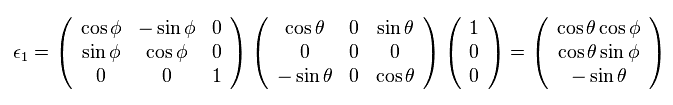This light is supposed to be scatterd by the electron at the origin toward z direction (= observer ).
So the polarization (= εout ) of scatterd light is in x-y plane.

This amplitude is given by inner product of "in" and "out" polarization.
Parameter Q is related to a square of amplitude.

(Eq.22)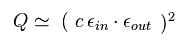Here, we suppose total intensity of incident light is "S".
And each intensity in ε1 and ε2 direction is supposed to be 1/2S.

(Eq.23)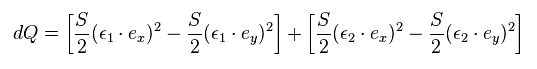Using "Q" of Eq.3 and summing all components of this incident light, we obtain parameter "Q" of the scatterd light, as shown in Eq.23.

(Eq.3)In the same way, parameter "U" of the scatterd light becomes
(Eq.24)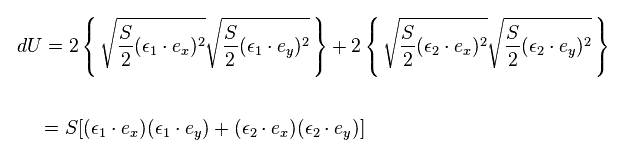From Eq.20 and Eq.21, we have
(Eq.25)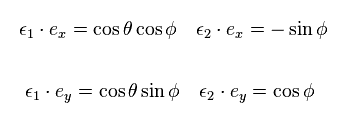Substituting Eq.25 into Eq.23 and Eq.24, we have
(Eq.26)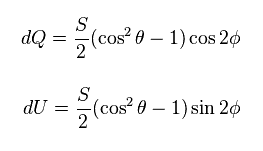where we use
(Eq.27)We add up incident lights from all directions.
So integrating Eq.26 over entire spherical surface,

(Eq.28)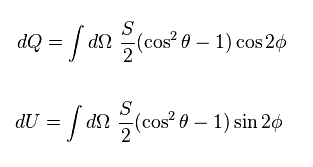where
(Eq.29)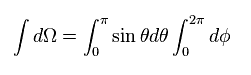Integrating Eq.28 over the surface, you find all these parameters become zero, because lights from different directions cancel each other out.
( This assumption is too simple ! )

## Influence of gravitational waves on B-mode polarization.

(Fig.22) Gravitaional waves stretch ( and contract ) space itself ?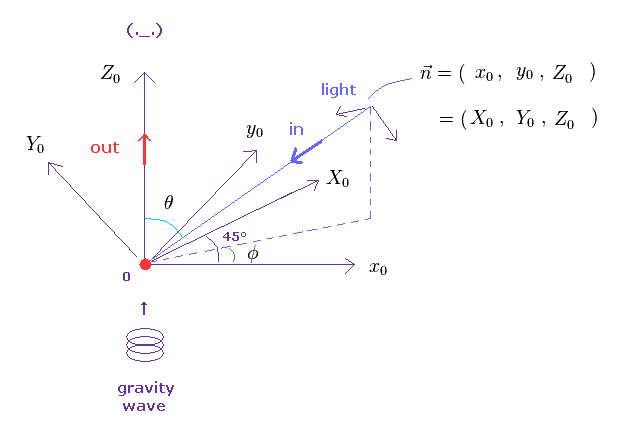Next we think about the case, in which some gravitational waves propagate in z direction.
This wave is expanding ( or contracting ) space itself in x-y plane.

The large "X" and "Y" is in x-y plane, and they are oriented at ±45 degree with respect to x axis.

(Fig.23) In x-y plane.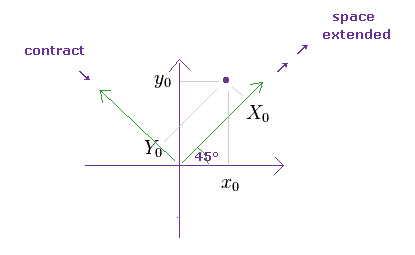Like the previous section, incident light comes towards the origin in "-n" direction.
Here we suppose, this gravitational waves extend the space in X direction, and contract it in Y direction, as follows,
(Eq.30)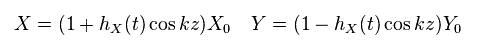From Fig.23, we have
(Eq.31)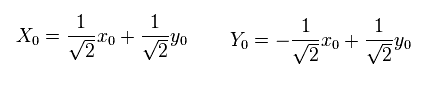And we suppose one light wavelength (= λ0 ) is given by
(Eq.32)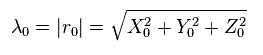This light wavelength is changed by space distortion by gravitational waves.
Substituting Eq.30 into Eq.32, we have

(Eq.33)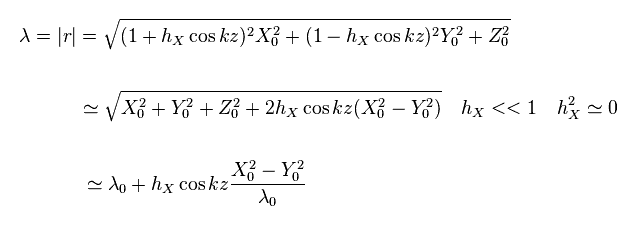Supposing hX is very small value, we neglect second-order effect of hX in Eq.33.
From Eq.33, the light frequenccy (= ν ) becomes

(Eq.34)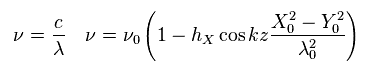Substituting Eq.31 into Eq.34
(Eq.35)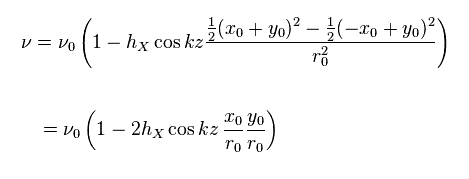where Eq.32 is used.
The change of light frequency means the change of temperature (= T ), as follows.

(Eq.36)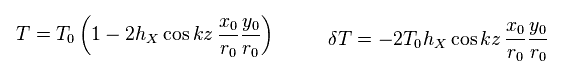According to Stefen-Boltzmann law, the radiated energy (= light intensity "S" ) is proportional to the fourth power of temperature T.

(Eq.37)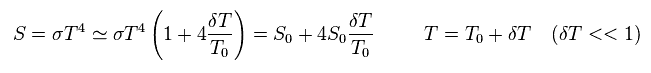Substituting Eq.36 into Eq.37, we have
(Eq.38)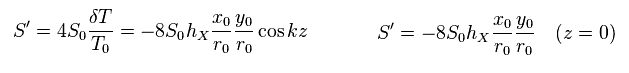Substituting x, y components of Eq.19 into S' of Eq.38,
(Eq.19)We have
(Eq.39)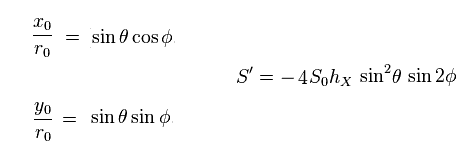Her we use the following results of the previous section.
(Eq.28)where
(Eq.29)Substituting Eq.39 and Eq.29 into Eq.28,

(Eq.40)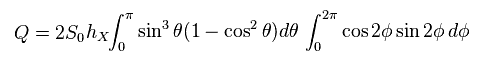where
(Eq.41)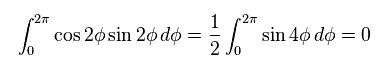So, parameter Q becomes zero.
On the other hand, U becomes

(Eq.42)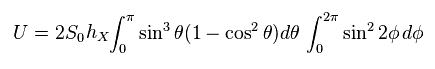where
(Eq.43)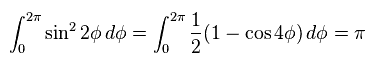and
(Eq.44)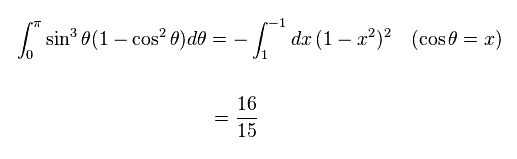As a result,
(Eq.45)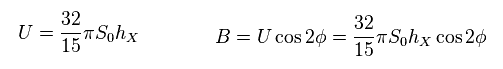From Eq.9, we find B-mode polarization is NOT zero by gravitational waves.
But as I said, it is impossible that faint CMB polarization 13.7 billion years ago remains as it is.

## Reason why pseudotensor is NOT real tensor.

(Fig.24) Equivalence principle → Gravtational waves are cancelled out !?On this page, we derive the following relation of Einstein energy momentum tensor (= T ),

(Eq.46)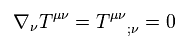The important point is that covariant derivative of general relativity is completely different from ordinary derivative ( see this site and this site. )

(Eq.47)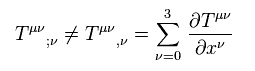In usual energy-momentum tesnor, the following conservation law is satisfied.
(Eq.48)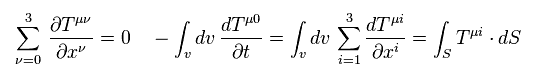But the covariant derivative (= ∇ ) of general relativity is very complicated,
(Eq.49)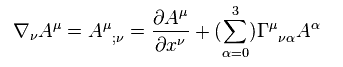As a result,
(Eq.50)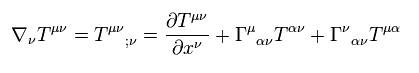where Christoffel symbol (= Γ ) is
(Eq.51)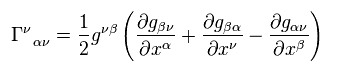where
(Eq.52)Here we use the following relation
(Eq.53)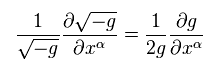(Eq.54)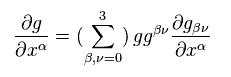Using Eq.54 in Eq.53, and comparing it with Eq.51,
(Eq.55)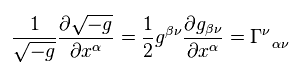Using Eq.55 in Eq.50,
(Eq.56)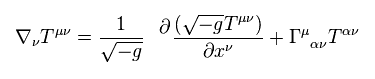As you see Eq.56, only the first term satisfies energy conservation law of tesnors.
So the energy and momentum of Einstein tensor are NOT conserved.

(Eq.57)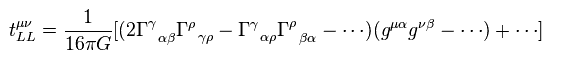As you see one example of pseudotensor of gravitational waves in Eq.57, this pseudotensor vanish in some coordinate such as locally inertial frame.
(= first order derivative with respect to metric tensor "g". )
So the interpretation of pulsar's decreased period by gravitational waves is meaningless.

## Spectrum of CMB.

(Eq.58)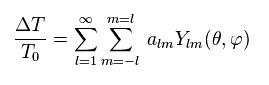In most textbooks, the distrubution of CMB temperature is expanded using spherical harmonics, as shown in Eq.58.

(Eq.59)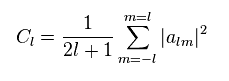Eq.59 is the coefficient representing temperature (= light intensity ).2014/3/25 updated. Feel free to link to this site.